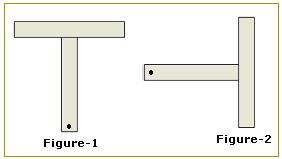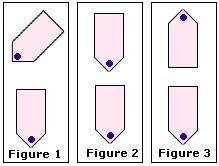## Definition Of Turn

Rotating a plane figure is called turn.

To rotate a plane figure.

### Examples of TurnFigure-2 is obtained by rotating the Figure-1.

### Solved Example on Turn

#### Ques: Which figure represents a turn?##### Choices:

A. Figure 1 and Figure 2
B. Figure 2 and Figure 3
C. Figure 1 and Figure 3
D. None of the above